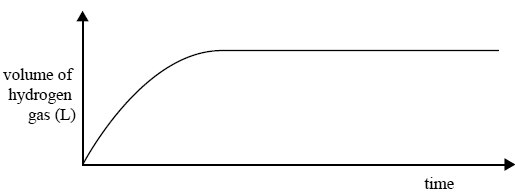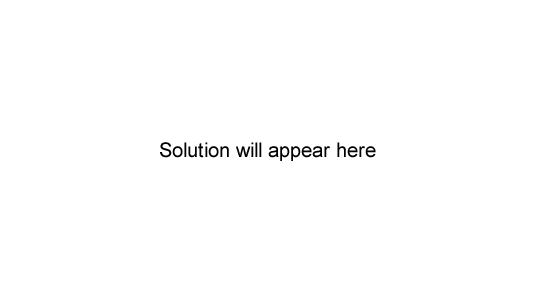Rate of reaction 2008 VCE A 2.0 g piece of magnesium ribbon was added to a known volume of 2.0 M hydrochloric acid. The volume of hydrogen gas produced during the reaction was measured and recorded. The graph below shows the result of this experiment.Write an equation for the reaction between magnesium and hydrochloric acid. Solution In a second experiment, 2.0 g of magnesium powder was added to the same volume of 2.0 M hydrochloric acid as used in the fi rst experiment. On the axes above, sketch the expected graph of volume of hydrogen against time for this second experiment. Give an explanation for the shape of your graph. Solution2) The following gaseous equilibrium is established at high temperatures in the presence of a finely divided nickel (Ni) catalyst. CH4(g) + H2O(g) => CO(g) + 3H2(g); ΔH = +206 kJ mol–1 The rate of a reaction generally increases with temperature. The factor that has the biggest effect on the increase in reaction rate is that with increasing temperature A. the activation energy of the reaction increases. B. the activation energy of the reaction decreases. C. the number of collisions between particles increases. D. the proportion of particles with high kinetic energy increases. Solution